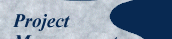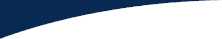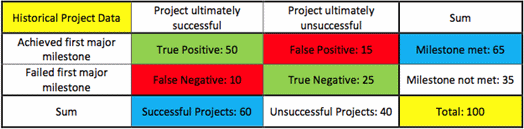## The Bayesian Paradigm, Part 2

Let's use our historical database of projects to put numbers into the cells:##### Figure 3: Historical Project Data

We normalize the numbers in the cells so that the total number of projects is 100; regardless of the actual number of projects, only the ratios matter. This normalization conveniently turns the numbers into percentages.

The ratio of green cells to red cells is one measure of the test, as red cells are bad predictions and green cells are good ones. The test gets it right only 75% of the time — the sum of the 50% and 25% in the green cells — indicating a weak predictor. Sixty percent of the projects — the number in the blue cell at the bottom — are successful. But the milestone is met 65% of the time; we see that in the blue cell at the right. The 65% success rate predicted by the first milestone does not equal the actual success rate of 60% because the test is imperfect.

What is the probability that the project will be successful if the first milestone is met? From the first line of the table, 65 projects met the first milestone and 50 were ultimately successful. We conclude that 50/65 or 77% of the projects that make their first milestone will succeed.

What is the probability that the project will fail if the first milestone is missed? From the second line, we have 35 that miss the milestone and 25 ultimately fail. So 25/35 or 71% of the projects that miss the first milestone will fail; only 29% will succeed.

If we believe that our project is typical of history with a predicted probability of success of 60%, then making the first milestone would boost it to 77% and missing would lower it to 29%. But when our initial estimate of the probability of success is different, say 55%, then we need to fold that initial estimate into the mix.

Every imperfect binary test can be characterized by two parameters. We obtain them from the 2x2 matrix:

e = (false negatives) / (true positives) = 10 / 50 = 0.20
f = (false positives) / (true negatives) = 15 / 25 = 0.60

We use these parameters to compute the weighted adjustment. The following statements about the parameters are different ways of saying the same thing. If you accept any one of them, you accept the other three as well:

1. These parameters characterize the strength of the test;
2. These parameters indicate the reliability of the new data;
3. These parameters quantify the predictive power of the milestone;
4. These parameters tell us how to weight the new information.

Let's calculate using Bayes' Theorem.